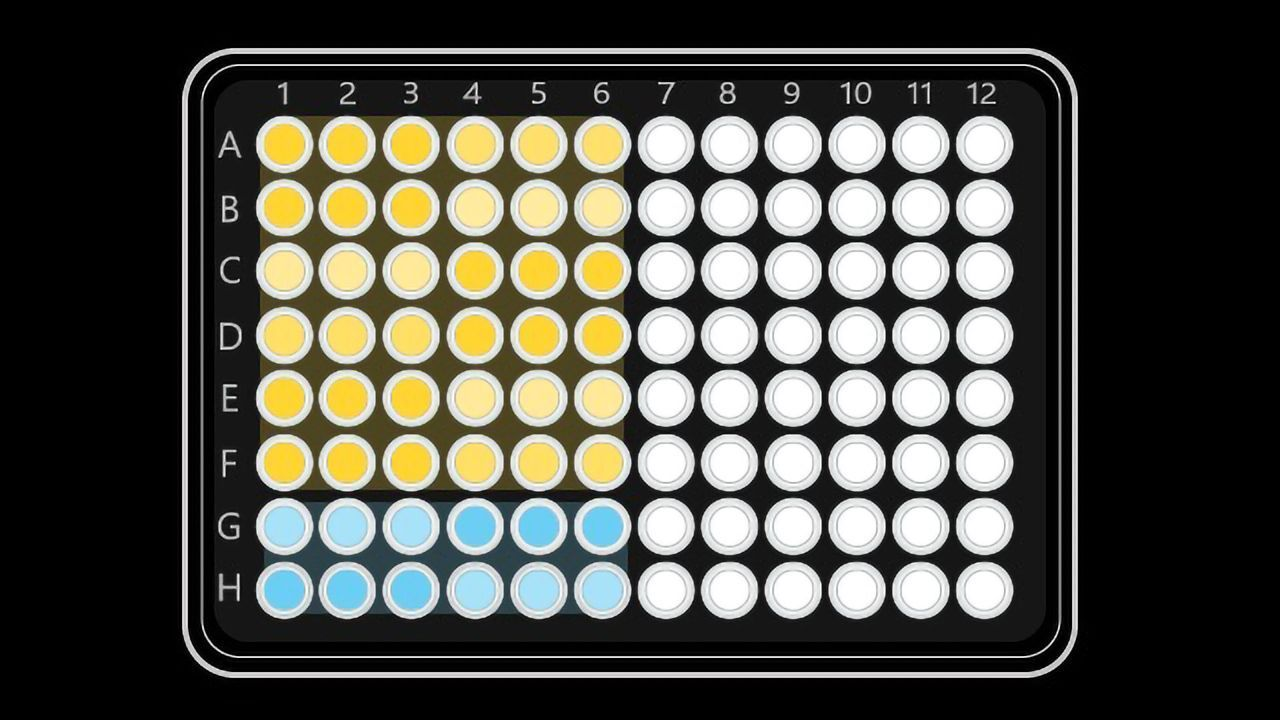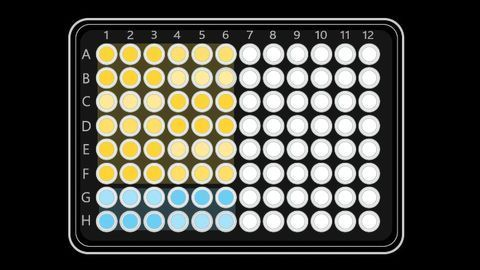We use cookies to provide you with a better experience. You can read our Cookie Policy here.Article

# Sensitivity vs SpecificityArticle

# Sensitivity vs Specificity

Complete the form below and we will email you a PDF version of "Sensitivity vs Specificity"

First Name*
Last Name*
Country*
Company Type*
Job Function*
Would you like to receive further email communication from Technology Networks?

Technology Networks Ltd. needs the contact information you provide to us to contact you about our products and services. You may unsubscribe from these communications at any time. For information on how to unsubscribe, as well as our privacy practices and commitment to protecting your privacy, check out our Privacy Policy

When developing diagnostic tests or evaluating results, it is important to understand how reliable those tests and therefore the results you are obtaining are. By using samples of known disease status, values such as sensitivity and specificity can be calculated that allow you to evaluate just that.

## What do sensitivity values tell you?

The sensitivity of a test is also called the true positive rate (TPR) and is the proportion of samples that are genuinely positive that give a positive result using the test in question. For example, a test that correctly identifies all positive samples in a panel is very sensitive. Another test that only detects 60 % of the positive samples in the panel would be deemed to have lower sensitivity as it is missing positives and giving higher a false negative rate (FNR). Also referred to as type II errors, false negatives are the failure to reject a false null hypothesis (the null hypothesis being that the sample is negative).

## What do specificity measures tell you?

The specificity of a test, also referred to as the true negative rate (TNR), is the proportion of samples that are genuinely negative that give a negative result using the test in question [Updated, January 25, 2022]. For example, a test that identifies all healthy people as being negative for a particular illness is very specific. Another test that incorrectly identifies 30 % of healthy people as having the condition would be deemed to be less specific, having a higher false positive rate (FPR). Also referred to as type I errors, false positives are the rejection of a true null hypothesis (the null hypothesis being that the sample is negative).

## Sensitivity vs specificity mnemonicSnNouts and SpPins is a mnemonic to help you remember the difference between sensitivity and specificity.

SnNout: A test with a high sensitivity value (Sn) that, when negative (N), helps to rule out a disease (out).

SpPin: A test with a high specificity value (Sp) that, when positive (P) helps to rule in a disease (in).

## How do I calculate sensitivity and specificity values?

An ideal test rarely overlooks the thing you are looking for (i.e., it is sensitive) and rarely mistakes it for something else (i.e. it is specific). Therefore, when evaluating diagnostic tests, it is important to calculate the sensitivity and specificity for that test to determine its effectiveness.

The sensitivity of a diagnostic test is expressed as the probability (as a percentage) that a sample tests positive given that the patient has the disease.

The following equation is used to calculate a test’s sensitivity:

Sensitivity = Number of true positives
(Number of true positives + Number of false negatives)

= Number of true positives
Total number of individuals with the illness

The specificity of a test is expressed as the probability (as a percentage) that a test returns a negative result given that the that patient does not have the disease.

The following equation is used to calculate a test’s specificity:

Specificity =     Number of true negatives

(Number of true negatives + number of false positives)

=     Number of true negatives
Total number of individuals without the illness

## Sensitivity vs specificity example

You have a new diagnostic test that you want to evaluate. You have a panel of validation samples where you know for certain whether they are definitely from diseased or healthy individuals for the condition you are testing for. Your sample panel consists of 150 positives and 400 negatives.

There are four things we will aim to clarify in this example:

1. What is the test's sensitivity? That is, how many diseased individuals does it correctly identify as diseased?
2. What is the test's specificity? That is, how many healthy individuals does it correctly identify as healthy?
3. What is the test's positive predictive value (PPV)? That is, what is the probability that a person returning a positive result is actually diseased?
4. What is the test's negative predictive value (NPV)?  That is, what is the probability that a person returning a negative result is actually healthy?

After running the samples through the assay, you compare your results to their known disease status and find:

True positives (test result positive and is genuinely positive) = 144

False positive (test result positive but is actually negative) = 12

True negatives (test result negative and is genuinely negative) = 388

False negative (test result negative but is actually positive) = 6

## Sensitivity vs specificity calculator

Want to calculate the sensitivity and specificity of your test? Add your results into our calculator here

## Sensitivity vs specificity table

Or, displayed in a contingency table:

 Genuinely Positive Genuinely Negative Row Total Test Positive 144 12 156 Test Negative 6 388 394 Column Total 150 400 550

Sensitivity = 144 / (144 + 6)
= 144 / 150
= 0.96
= 96 % sensitive

Specificity = 388 / (388 + 12)
= 388 / 400
= 0.97
= 97 % specific

## Are sensitivity and specificity the same as the positive predictive value (PPV) and negative predictive value (NPV)?

In short, no, although they are related. The positive predictive value (PPV) is the probability that a subject/sample that returns a positive result really is positive. The negative predictive value (NPV) is the probability that a subject/sample that returns a negative result really is negative. This sort of information can be very useful for discussing results with a patient for example, evaluating the reliability of any test they may have had. The same values used to calculate the sensitivity and specificity are also used to calculate the positive and negative predictive values. One way to look at it is that the sensitivity and specificity evaluate the test, whereas the PPV and NPV evaluate the results.

The positive predictive value is calculated using the following equation:

PPV = Number of true positives

(Number of true positives + Number of false positives)

= Number of true positives
Number of samples that tested positive

The negative predictive value is calculated using the following equation:

NPV = Number of true negatives

(Number of true negatives + Number of false negatives)

= Number of true negatives
Number of samples that tested negative

Using the values from the example above:

PPV = 144 / (144 + 12)
= 144 / 156
= 0.923076923… = 92 %

NPV = 388 / (388 + 6)
= 388 / 394
= 0.984771573… = 98 %

So, if a test result is positive, there is a 92 % chance it is correct, if it is negative there is a 98 % chance it is correct.

The complementary value to the PPV is the false discovery rate (FDR), the complementary value of the NPV is the false omission rate (FOR) and equates to 1 minus the PPV or NPV respectively. The FDR is the proportion of results or “discoveries” that are false. The FOR is the proportion of false negatives which are incorrectly rejected. Essentially, the higher the PPV and NPV are, the lower the FDR and FOR will be - which is good news for the reliability of your test results.

## How should I balance sensitivity with specificity?

Where results are given on a sliding scale of values, rather than a definitive positive or negative, sensitivity and specificity values are especially important. They allow you to determine where to draw cut-offs for calling a result positive or negative, or maybe even suggest a grey area where a retest would be recommended. For example, by putting the cutoff for a positive result at a very low level (blue dashed line), you may capture all positive samples, and so the test is very sensitive. However, this may mean many samples that are actually negative could be regarded as positive, and so the test would be deemed to have poor specificity. Finding a balance is therefore vital for an effective and usable test.Using a receiver operating characteristic (ROC) curve can help to hit that sweet spot and balance false negatives with false positives. However, the context is also important as to whether false negatives are less problematic than false positives, or vice versa. For example, if it is imperative that all positives are identified – for example, in a matter of life and death, then you may be willing to tolerate a higher number of false positives to avoid missing any. Here, false positives can be screened out further down the line.

## What is a ROC curve?

A ROC curve is a graphical representation showing how the sensitivity and specificity of a test vary in relation to one another. To construct a ROC curve, samples known to be positive or negative are measured using the test.

The TPR (sensitivity) is plotted against the FPR (1 - specificity) for given cut-off values to give a plot similar to the one below. Ideally a point around the shoulder of the curve is picked which both limits false positives whilst maximizing true positives.A test that gave a ROC curve such as the yellow line would be no better than random guessing, pale blue is good, but a test represented by the dark blue line would be excellent. It would make cutoff determination relatively simple and yield a high true positive rate at very low false positives rate – sensitive and specific.

Correction: The article erroneously stated the definition  of "specificity" as "the proportion of samples that test negative using the test in question that are genuinely negative," this was updated on January 25, 2022 to define it correctly as "the proportion of samples that are genuinely negative that give a negative result using the test in question."

Meet the AuthorKaren Steward PhD
Senior Science Writer